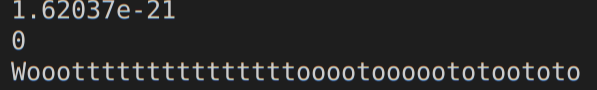# Negative value get from Gaus!

ROOT Version: 6.20.04
Platform: Linux Mint 19
Compiler: g++

Hello everybody!
I faced the following problem while working on an application with root classes:Is it possible to avoid this bug? I don’t understand type of gotten value, because I don’t convert this to positive numeric value…

code from loop :
cout << gRandom->Gaus(spectr[t],sqrt(spectr[t])) << endl;
cout << (gRandom->Gaus(spectr[t],sqrt(spectr[t])) > 0) << endl; //val from output > zero!
cout << “Woootttttttttttttttooootooooototoototo\n”;

my output:
1.62037e-21
0
Woootttttttttttttttooootooooototoototo

Hi,
I am not sure I have understood your problem. `gRandom->Gaus(m, s)` returns a random number distributed with a Gaussian distribution with mean =m and sigma=s. Depending on m and s values you have for sure negative values.

Lorenzo

Thanks for your reply. But we know that Gaussian distribution gives probability which can not be negative. Essentially first and third lines in my code are needless. But when we compare random number from Gauss distribution in the second line, result is unexpected.

Why the result of this line `(gRandom->Gaus(spectr[t],sqrt(spectr[t])) > 0) == false` is unexpected ?

Do you suppose that gRandom->Gaus(spectr[t],sqrt(spectr[t])) simply equal zero? I don’t suggest this, but it is really possible…

zero is unlikely but can happen and certainly it can be < 0. All depends on the parameters that you pass, `spectr[t].`

Could you please give an example of parameters (mean val, deviation) that lead to a negative value of the Gaussian distribution?

I could represent complex parameters satisfying this condition, but how can real parameters be such?

As I said before we are talking about Gaussian distributed number (those returned by gRandom->Gaus) and not distribution values (those evaluated by `TMath::Gaus`).
For example:

``````for (int i = 0; i < 10; ++i) cout << gRandom->Gaus(0,1) << endl;
``````

Thank you very much! I realized.

This topic was automatically closed 14 days after the last reply. New replies are no longer allowed.